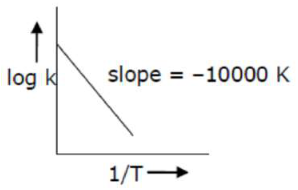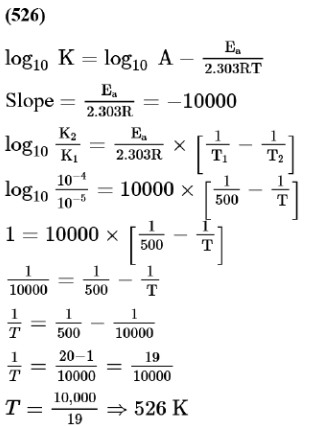# Solve the followingQuestion:

For the reaction, $a A+b B \rightarrow c C+d D$, the plot of $\log k v s \frac{1}{T}$ is given below:The temperature at which the rate constant of the reaction is $10^{-4} \mathrm{~s}^{-1}$ is K. [Rounded off to the nearest integer) [Given: The rate constant of the reaction is $10^{-5} \mathrm{~s}^{-1}$ at $500 \mathrm{~K}$ ]

Solution: